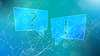# TechTopics No. 46

Selection of current transformer (CT) ratio in medium-voltage control

Selection of the appropriate current transformer (CT) ratio for motor controllers is relatively straight-forward, but must consider a number of factors. The CT ratio is selected to provide proper overload protection to the machine. Short-circuit protection considerations are not a factor, as the short-circuit protection in a NEMA Class E2 controller is provided by the current-limiting fuses (refer to TechTopics No. 45). The application procedure that Siemens uses to select the CT ratio is as follows:

a. Multiply motor full-load current (FLA) x 1.5. Select the standard CT primary rating closest to the 1.5 x FLA figure as a first approximation. Example: motor FLA = 72 A. 72 x 1.5 = 109 A, select 100:5 CT.

b. Calculate the CT secondary current at motor FLA. Example: 73 A/(100/5) = 3.65 A.

c. Calculate the CT secondary current at motor locked-rotor current (LRA). Example: motor LRA = 650 percent FLA, (6.5 x 73 A)/(100/5) = 23.7 A.

d. The CT secondary current at motor FLA (refer to step b) should be no higher than 4.0 A.

e. The CT secondary current at motor LRA (refer to step c) should be no higher than 24.0 A.

f. If the conditions in steps d and e are both satisfied, and the CT secondary wire length (total loop distance) is under

20 feet, and the wire size is at least #14AWG, then the selected CT ratio is ok.

g. If the conditions in either steps d or e are violated, the engineer must specifically evaluate the accuracy of the CT to assure that the CT will have the necessary accuracy for the application. This requires calculation of secondary burden, including the burden of the protective device, in order to make a decision.

Since the individual evaluation per step g is pretty rare, it will not be discussed further in this example.

Siemens methodology is simplified (very conservative), so that the maximum number of applications will be met by the standard CT selected from steps a through f, and it is seldom necessary to perform a more extensive individual evaluation per step g. Siemens methodology assumes all worst-case conditions (e.g., historic mechanical relays, multiple devices in CT circuit, longest wire circuit in any controller configuration, etc.).

Since the base calculation starts from 150 percent of the motor FLA, this seldom yields a CT ratio of less than 125 percent of the motor FLA. In fact, step d essentially requires that the CT primary current rating be at least 125 percent of motor FLA. Step e is used to avoid issues when the motor LRA is higher than 600 percent of the full load current (FLA).